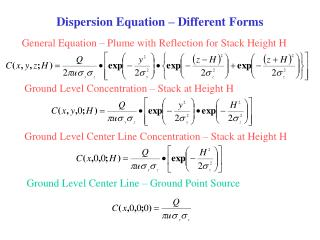# Dispersion Equation – Different Forms - PowerPoint PPT PresentationDownload PresentationDispersion Equation – Different Forms

Dispersion Equation – Different Forms
Download Presentation## Dispersion Equation – Different Forms

- - - - - - - - - - - - - - - - - - - - - - - - - - - E N D - - - - - - - - - - - - - - - - - - - - - - - - - - -
##### Presentation Transcript

1. Dispersion Equation – Different Forms General Equation – Plume with Reflection for Stack Height H Ground Level Concentration – Stack at Height H Ground Level Center Line Concentration – Stack at Height H Ground Level Center Line – Ground Point Source

2. Calculation of Effective Stack Height Note that H = hs + h, where h is the stack rise. Stack rise is dependant on stack characteristics, Meteorology, and physico-chemical nature of effluent. * Carson-Moses Equation: * Holland Formula: * Concawe Formula: Where

3. Preferred Equation for Stack Rise

4. Wind Velocity U for the Model • U = f(z) given by (U/U1) = (z/z1)p, where p depends on on atmospheric stability. • Appropriate value of U for dispersion model is the mean value through the plume. • If mean U is unavailable, use appropriate U at stack height. In most cases only U10m is available- then correct for U at stack height using above equation. • If no mention of height of measurement of U is made use U as mean. If measured height is specified for U, then correct for it to get U at stack height.

5. Estimating Emission Rate Q for Various Scenarios: (Ref: A Kumar, Pollution Engineering, p 52, February (1996) Accidental Release of Low volatile liquid from a tank on ground: Accidental Release of Highly Volatile Liquid from Tank on Ground: Accidental Release of Heavier-than-air gas from a tank on ground:

6. Concentration Isopleths Concentration / g.m-3 0 0 Downwind distance / meters Concentration isopleths

7. Model of flow around a sharp edged 3-D building in a deep boundary layer Incident wind profile Reattachment lines on sides and roof Lateral edge and elevated vortex pair Cavity zone Turbulent wake Mean cavity reattachment line Separation lines and Horseshoe vortex system Length L, Width W, Height H

8. Cavity Length for Short and Long Buildings Short Buildings: Long Buildings: Note: For long buildings, independent of L/H. Maximum height of cavity:

9. Effective stack height with buildings 1. Correction for stack-induced downwash: 2. Building induced downwash: - stack induced downwash is first determined, then building effect is appended Let b be the smaller of H or W If hs’ > H + 1.5 b,  hs” = hs’ If hs’ < H  hs” = hs’ – 1.5 b If H <hs’  hs” = 2hs’ – (H + 1.5 b) 3. Entrained plumes: If hs” > b, plume remains aloft. If hs” < b, plume trapped in cavity and treat as ground level source with area b2.

10. Effective stack height with buildings – continued. 4. Plume buoyancy effect: If plume is air (mostly) and Tplume same as Tamb hs’ = hs” • If not calculate density difference:  = (Me/Ma)(Ta/Te) –1 • Where a is air and e is effluent. • < 0  standard procedure for hs • > 0  other procedures used. 5. Downwind concentration far from the stack: Use usual formula from dispersion model.

11. Concentration in Cavity Wake. More appropriate (takes bldg. dim. into account):

12. Concentration immediately downwind of wake cavity For trapped plumes consider source as ground level: For other cases: where

13. Obtaining y and z

14. Stability Classes Note that both y and z can be obtained from Tables 4-1 and 4-2 of the textbook (Wark, Warner and Davis: Air Pollution, 3rd edition)

15. EPA Air Quality and Dispersion Models http://www.epa.gov/scram001/tt22.htm